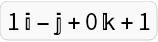Function Repository Resource:

Quaternion

Represent a quaternion object

Contributed by: Wolfram Staff
 ResourceFunction["Quaternion"][a,b,c,d] returns the quaternion number a+bⅈ+cⅉ+d𝕜.

Details and Options

Quaternions have the form a+b+c+d𝕜 where a, b, c and d are real numbers.
The symbols , and 𝕜 are multiplied according to the rules 2=2=𝕜2=ⅈ ⅉ 𝕜=-1.
Quaternions are an extension of the complex numbers and work much the same except that their multiplication is not commutative. For instance, ⅈⅉ=-ⅉⅈ.
Because of the similarities between quaternions and complex numbers, this function imitates the Wolfram Languages treatment of complex numbers in many ways.
To provide a clear distinction between quaternions and complex numbers all quaternions should be entered using the form ResourceFunction["Quaternion"][a,b,c,d] where a, b, c and d are real numbers.
Only limited support is offered to the symbolic form a+Ib+J c+Kd.

Examples

Basic Examples (3)

Get the quaternion 1+2ⅈ+3ⅉ+4𝕜:

 In:=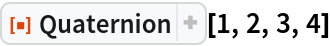Out=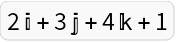In:=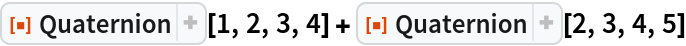Out=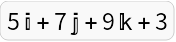Use NonCommutativeMultiply (**) to multiply quaternions:

 In:=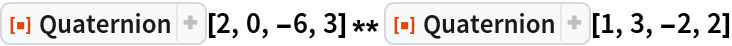Out=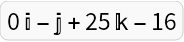This multiplication is noncommutative:

 In:=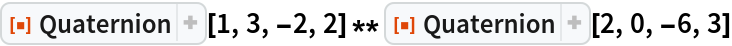Out=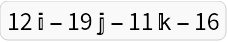Properties and Relations (7)

In the conjugate of a quaternion, all the signs of the nonreal components are reversed:

 In:=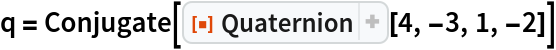Out=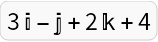The sign of a quaternion is defined in the same way as the sign of a complex number. It is the “direction” of the quaternion:

 In:=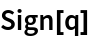Out=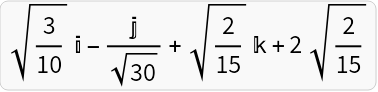Get the standard Euclidean length:

 In:=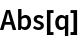Out=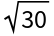The exponential of a quaternion can be quite complicated:

 In:=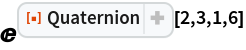Out=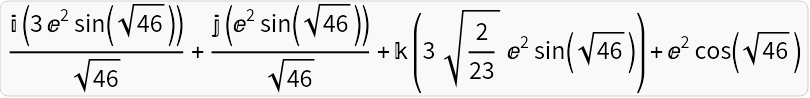Just as with complex numbers, it is important to beware of branch cuts:

 In:=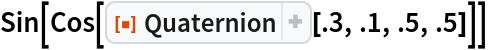Out=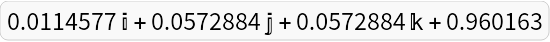A four‐dimensional analog of de Moivre’s theorem is used for calculating powers of quaternions:

 In:=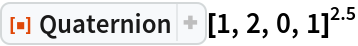Out=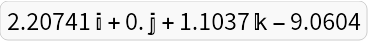Round for quaternions returns a Quaternion in which either all components are integers or all components are odd multiples of 1/2:

 In:=Out=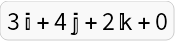A quaternion is even if its norm is even:

 In:=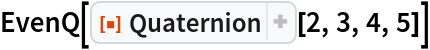Out=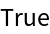In:=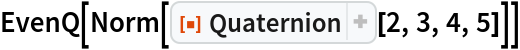Out=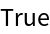Just as with complex numbers, the quaternion Mod works:

 In:=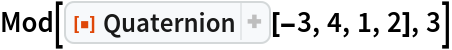Out=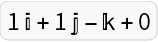You can specify a quaternion as the modulus:

 In:=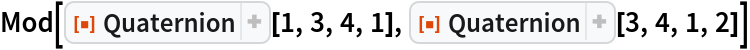Out=